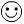Trichotomy Property

The Trichotomy Property means that when you are comparing 2 numbers, one of the following must be true: the first number is bigger than the second number, the first number is smaller than the second number or the two numbers are equal. This is a common sense property with a hard nameLook how easy this is:

Example 1: given two numbers 5 and 7

• 5 is less than 7     5 < 7

• 5 is equal to 7       5 = 7

• 5 is greater than 7     5 > 7

• Which statement is true? 5 is less than 7.

Can more than one statement be true? No. 5 can not be equal to or greater than 7.

Example 2: Todd has 4 apples and Jorge has 10 apples. Which statement is true?

• Todd has more apples than Jorge.
• Todd has the same amount of apples as Jorge.
• Todd has fewer apples than Jorge.

Example 3: Amanda scored more points in basketball than Lauren. What else could we say about the scores?

• Lauren could NOT have scored more than Amanda
• Lauren could NOT have scored the same amount as Amanda.

Let's sum this up by using variables (letters instead of numbers). Only one statement can be true about any 2 numbers a and b.

a < b     a = b     or    a > b

One last example: Alex is shorter than Barry. It follows than Barry is taller than Alex and they cannot be the same height.
Math
algebra
Inverse Properties of Addition and Multiplication
Distributive Property

To link to this Trichotomy Property page, copy the following code to your site: